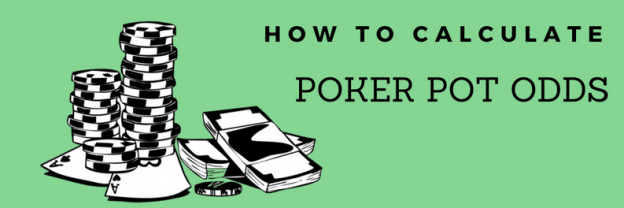# How to Calculate Poker Pot Odds & Percentages

Using mathematics and probability in poker is one of the most useful (yet underused!) skills for earning profit over the long run.

Adding these skills to your arsenal will help to support the decision-making process. For instance, adding certain calculations to your game will enable you to determine whether making a call, raise or fold in any given situation is the most profitable play in the long run (also referred to as +EV). While there are a few different calculations which can help you to elevate your game, one of the most fundamental is factoring in pot odds.

This article aims to explain what pot odds are, how they work, and how to incorporate them into your game.

## What are poker pot odds?

Pot odds are the immediate odds you are being offered when you call a bet in poker. This is often called the “reward-to-risk” ratio and it calculates how much you stand to win immediately in relation to what you have to risk by calling a bet.

In the case of pot odds, the math is telling the player whether a call, raise or fold is a mathematically sound play, given the risk-to-reward ratio.

Take, for example, a hand in which you have contributed a huge proportion of your stack pre-flop and you are now heads-up. You have a made hand and are convinced that your opponent hasn’t connected. Now, if your opponent was to put in a bet on the river which was a mere fraction of the overall pot, you would call, right? Of course. This is because the “risk-to-reward” ratio is telling you that it makes sense to contribute just a few more chips for the chance to win the whole pot. Let’s dig a little deeper and find out exactly what this calculation looks like.

## How to calculate pot odds?

Pot odds = [pot size]:[amount to call] where pot size includes any and all bets on the current street (pre-flop, flop, turn or river) as well as the amount in the middle. The final sum is expressed as a ratio.

### Pot odds example

The pot is £400 and there is a £200 bet in front of you. You would follow the steps below to determine your pot odds:

Pot odds = [pot size]:[amount to call]

= [£400 + £200]:[£200]

= £600:£200 = 3:1

Pot odds = 3:1

## What do pot odds really mean?

So what do 3:1 pot odds really mean? Well, in a nutshell, it means that you are required to put 25% more money into the pot if you decide to call. This is because the final pot size would be £800 and our call is £200 (800/200 is 25%).

We use pot odds to ensure we only call bets when we are getting good pot odds. While it’s best to use pot odds in conjunction with implied odds (we’ll cover this another day), equity and position, you can still use this calculation to see whether a call is preferable or not. As a rule of thumb, the bigger your pot odds, the better price you have for calling. In the above example, for example, it would be far more attractive to call a bet that gives you odds of 4/1 or 5/1.

### Scenario

Let’s do a simple pot odds exercise to help you learn how to quickly determine pot odds ratios and percentages.

### Question

Villain bets £50 into a £50 pot and it is your turn to act. What are your pot odds ratio and percentage?

If you said 2:1 or 33% give yourself a big pat on the back. Here’s how it is calculated:

### Pot odds calculation

Pot odds = [pot size]:[amount to call]

Pot size = £50 pot + £50 bet = £100]

= £100:£50 = 2:1

2:1 pot odds ratio

### Pot odds % calculation

Pot odds – call size/pot size

Pot size = £50 + £50 + £50 call = £150

= £50 / £150 = 1/3 = 33%

33% pot odds

## How to calculate hand percentages

Unlike pot odds, it can be extremely difficult to calculate hand percentages during a fast-moving game. This is often frustrating for poker players when they’re trying to make a quick decision or wondering what went wrong.

Enter, the Grosvenor Casinos Poker Calculator. Using the “Monte Carlo” algorithm, this awesome tool estimates the percentage of making a hand by simulating what could happen in any given scenario. The calculator then runs all the possible outcomes based on what each player has at the time.

Try out the Poker Calculator for yourself to get yourself ready for our upcoming Goliath tournament.